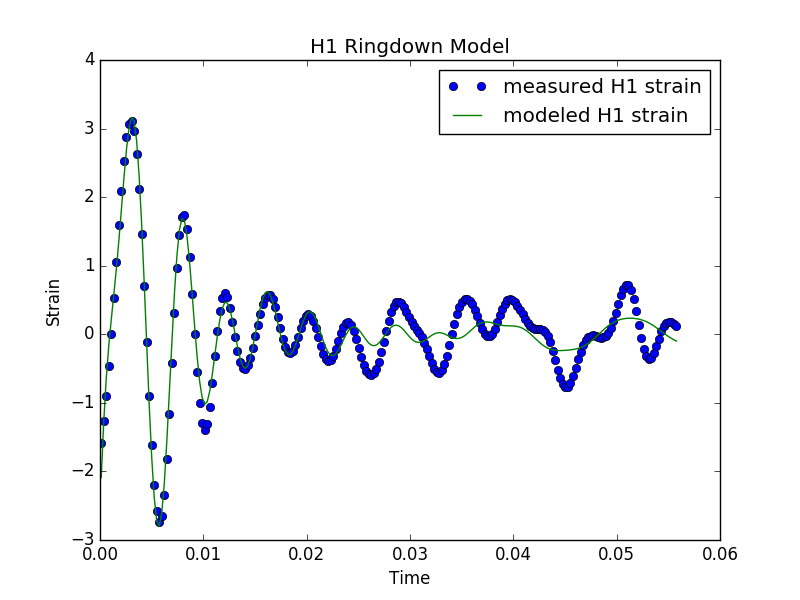dummy-link

6

0

0

1

# Varpro: Variable Projection for Nonlinear Least Squares Problems

Model fitting is often approached as an optimization problem where the sum of the model errors are minimized by optimizing the model parameters. If some of the model parameters are non-linear, then a non-linear optimization algorithm must be used. This can be inefficient if some of the parameters are linear.

The Varpro algorithm recasts the problem so that only the nonlinear parameters need to be considered by the nonlinear optimizer. For more details see the references below and the embedded docs in the source code.

This Julia code is a translation and extension the of the Matlab code that can be found here. The extensions involve handling complex inputs and a complex model (although the optimization objective function remains real since the objective is essentially the L2 norm of the residual (error) vector).

## Usage

The best way to learn how to use varpro is to read reference . The usage in Julia differs somewhat from the MATLAB version. With this version, we first set up a FitContext by calling the constructor as in the following

``````ctx = FitContext(y, t, w, x_init, n, ind, f_exp, g_exp)
``````

All of these are required parameters. The vector y is the data we wish to fit sampled at the times t. The vector w is a weight vector for selectively weighting the data. The vector x_init is the starting guess. Note that both y and x_init can be either real or complex, but they both must share the same type. The integer n is the number of basis functions (ie the number of linear parameters). The matrix ind specifies the structure of the dphi matrix (see ). The functions f_exp and g_exp calculate the phi and dphi matrices.

The following is a complete example of fitting the H1 strain ringdown values of the recently discovered gravity wave GW150914 .

``````using Varpro
using PyPlot
using DelimitedFiles

function f_exp(alpha, ctx)
for j = 1:ctx.n
for i in 1:ctx.m
ctx.phi[i, j] = exp(-alpha[j] * ctx.t[i])
end
end
ctx.phi
end

function g_exp(alpha, ctx)
for i in 1:ctx.m
ctx.dphi[i, :] = -ctx.t[i] * ctx.phi[i, :]
end
ctx.dphi
end

""" Fit n complex exponentials to the measured data """
function exp_fit(n, y, t)
w = ones(length(t))
ind = [collect(1:n)'; collect(1:n)']
x_init = complex.(0.1*rand(n), 2.0*rand(n))
ctx = FitContext(y, t, w, x_init, n, ind, f_exp, g_exp)
(alpha, c, wresid, resid_norm, y_est, regression) = varpro(ctx)
end

function main()
h1 = readdlm("h1_whitened.txt")
t = h1[:, 1]
y = complex.(h1[:, 2])  # must be complex to match x_init
x, c, r, r_norm, y_est, reg = exp_fit(6, y, t)
println("Norm of residual error: ", r_norm)
plot(t, real(y), "o", label="measured H1 strain")
plot(t, real(y_est), label="modeled H1 strain")
xlabel("Time")
ylabel("Strain")
title("H1 Ringdown Model")
legend(loc="upper right")
savefig("modeled_GW150914_strain.png")
end

main()
``````

The above code produces the following figure:## Limitations

Only supported in Julia 0.7 (dev)

## References

 Golub, G.H., Pereyra, V.: "The differentiation of pseudoinverses and nonlinear least squares problems whose variables separate". SIAM Journal on Numerical Analysis 10, pp 413-432 (1973)

 Golub, G.H., Pereyra, V.: "Separable nonlinear least squares: The variable projection method and its applications". Inverse Problems 19 (2), R1–R26 (2003)

 Pereyra, V., Scherer, eds: "Exponential Data Fitting and its Applications" Bentham Books, ISBN: 978-1-60805-048-2 (2010)

 Dianne P. O'Leary, Bert W. Rust: "Variable projection for nonlinear least squares problems". Computational Optimization and Applications April 2013, Volume 54, Issue 3, pp 579-593 Available here

 B. P. Abbott el. al. "ASTROPHYSICAL IMPLICATIONS OF THE BINARY BLACK HOLE MERGER GW150914" The Astrophysical Journal Letters, Volume 818, Number 2

04/21/2016

about 1 year ago

30 commits